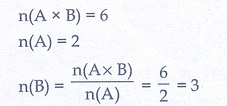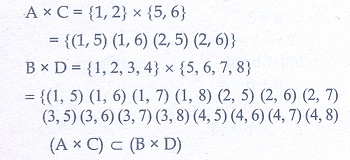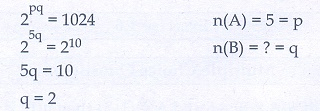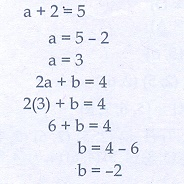# Chapter 1: Relation and Function - Online Test

Q1. If  n(A× B) = 6 and A = {1, 3} then n(B) is
Explaination / Solution:Q2. A = {a,b, p},  B = {2, 3}, = {p,q,r,s} then n[(A C B] is
Explaination / Solution:Q3. If  A = {1, 2}, B = {1, 2, 3, 4}, = {5, 6} and D = {5, 6, 7, 8} then state which of the following statement is true.
Explaination / Solution:Q4. If there are 1024 relations from a set A = {1, 2, 3, 4, 5} to a set B, then the number of elements in B is
Explaination / Solution:Q5. The range of the relation R = {(x, x2 ) | x is a prime number less than 13} is
Explaination / Solution:Q6. If the ordered pairs (a + 2, 4) and (5, 2a + b) are equal then (a,b) is
Explaination / Solution:Q7. Let n(A) = and n(B) = n then the total number of non-empty relations that can be defined from A to B is
Explaination / Solution:Q8. If {(a, 8),(6,b)} represents an identity function, then the value of a and b are respectively
Explaination / Solution:
No Explaination.

Q9. Let A = {1, 2, 3, 4} and B = {4, 8, 9,10}. A function f: A B given by= {(1, 4),(2, 8),(3, 9),(4,10)} is a
Explaination / Solution:
No Explaination.

Q10. If f (x) = 2x2 and g(x) = 1/3x then f o g is
Explaination / Solution: